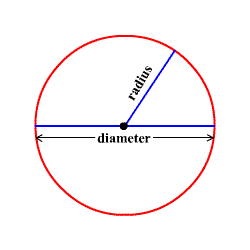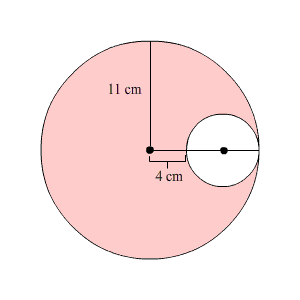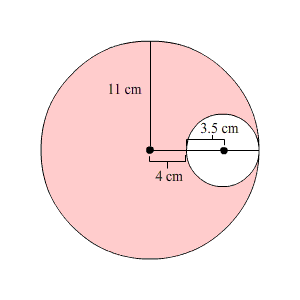# Circles: Area

A circle is the set of all points in a plane at a given distance (called the radius ) from a given point (called the center.)

A line segment connecting two points on the circle and going through the center is called a diameter of the circle.Clearly, if $d$ represents the length of a diameter and $r$ represents the length of a radius, then $d=2r$ .

The circumference $C$ of a circle is the distance around the outside. For any circle, this length is related to the radius $r$ by the equation

$C=2\pi r$

where $\pi$ (pronounced " pi ") is an irrational constant approximately equal to $3.14$ .

The area of a circle is given the formula

$A=\pi {r}^{2}$ .

Example 1:

What is the area of a circular table with diameter $6$ ft?

Given that, the diameter of the circular table is $6$ ft.

So, the radius of the circular table is half of the diameter. That is, radius is $3$ ft.

Use the formula for area of a circle, $A=\pi {r}^{2}$ , where $r$ is the radius of the circle.

Substitute $3$ for $r$ in the formula.

$\begin{array}{l}A=\pi {\left(3\right)}^{2}\\ \text{\hspace{0.17em}}\text{\hspace{0.17em}}\text{\hspace{0.17em}}\text{\hspace{0.17em}}\text{\hspace{0.17em}}=\pi \left(9\right)\\ \text{\hspace{0.17em}}\text{\hspace{0.17em}}\text{\hspace{0.17em}}\text{\hspace{0.17em}}\text{\hspace{0.17em}}\approx 28.26\end{array}$

Therefore, the area of the circular table is about

Example 2:

What is the area of the shaded region in the figure shown?It's clearly marked that the larger circle has a radius $11$ cm. So, its area is

What about the smaller circle? Well, the distance from the center to edge is $11$ cm; the diameter of the smaller circle is $11-4=7$ cm. So, the radius of the smaller circle is $3.5$ cm.To get the area of the shaded region, subtract the area of the smaller circle from the area of the larger circle.

$379.94-38.47=341.47$